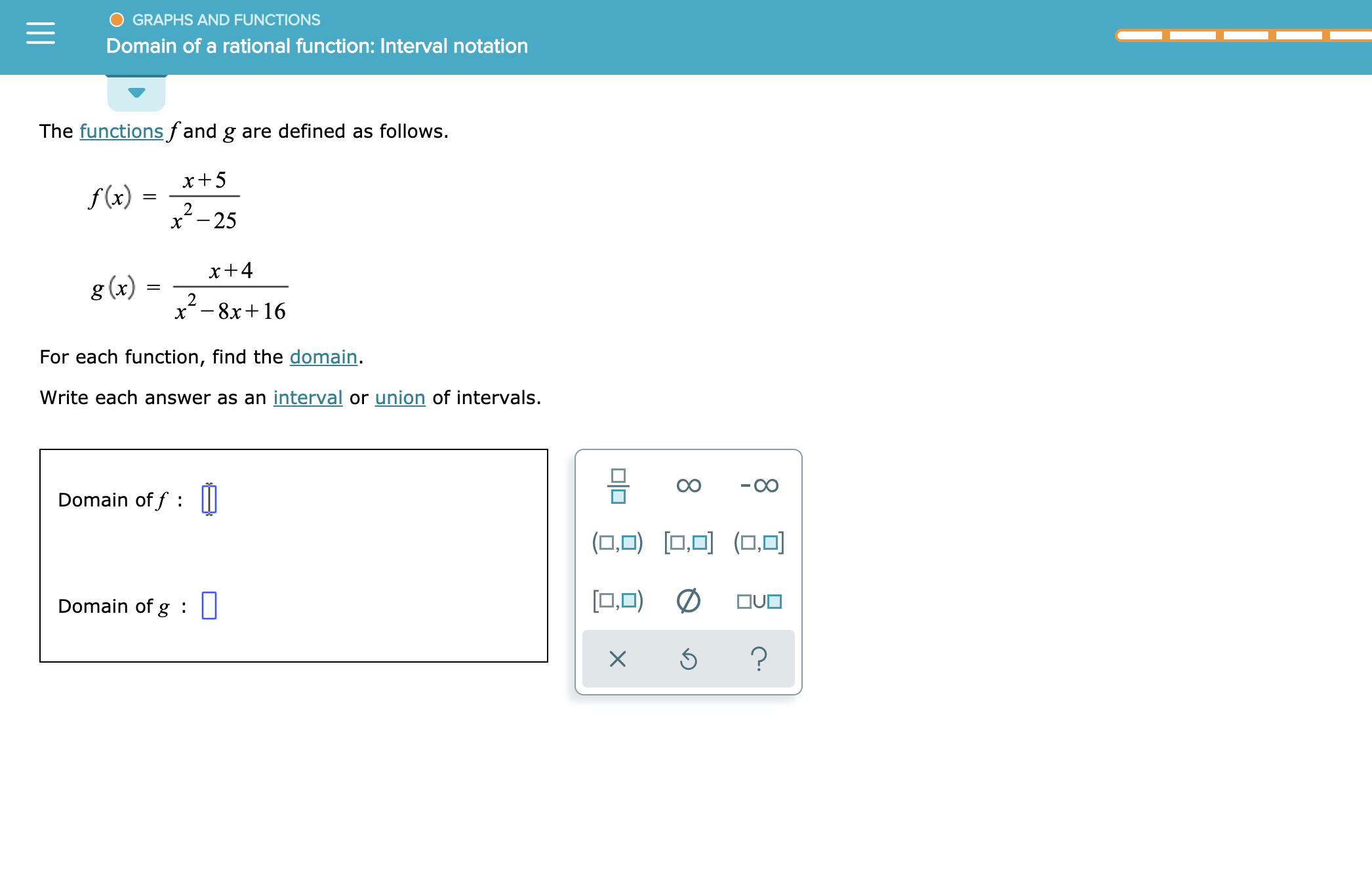# GRAPHS AND FUNCTIONSDomain of a rational function: Interval notationThe functionsfand g are defined as follows.x+5f(x)=2x-25x+ 4g (x)2x-8x16For each function, find the domain.Write each answer as an interval or union of intervals.Domain of f:1,0)OUODomain of g||X

Question

see attachmenthelp_outlineImage TranscriptioncloseGRAPHS AND FUNCTIONS Domain of a rational function: Interval notation The functionsfand g are defined as follows. x+5 f(x) = 2 x-25 x+ 4 g (x) 2 x-8x16 For each function, find the domain. Write each answer as an interval or union of intervals. Domain of f : 1,0) OUO Domain of g || X fullscreen
check_circleExpert Solution
Step 1

For a Rational function R(x) = p(x) / q(x) ,lowest form, domain is giv...

### Want to see the full answer?

See Solution

#### Want to see this answer and more?

Solutions are written by subject experts who are available 24/7. Questions are typically answered within 1 hour*

See Solution
*Response times may vary by subject and question
Tagged in

### Functions